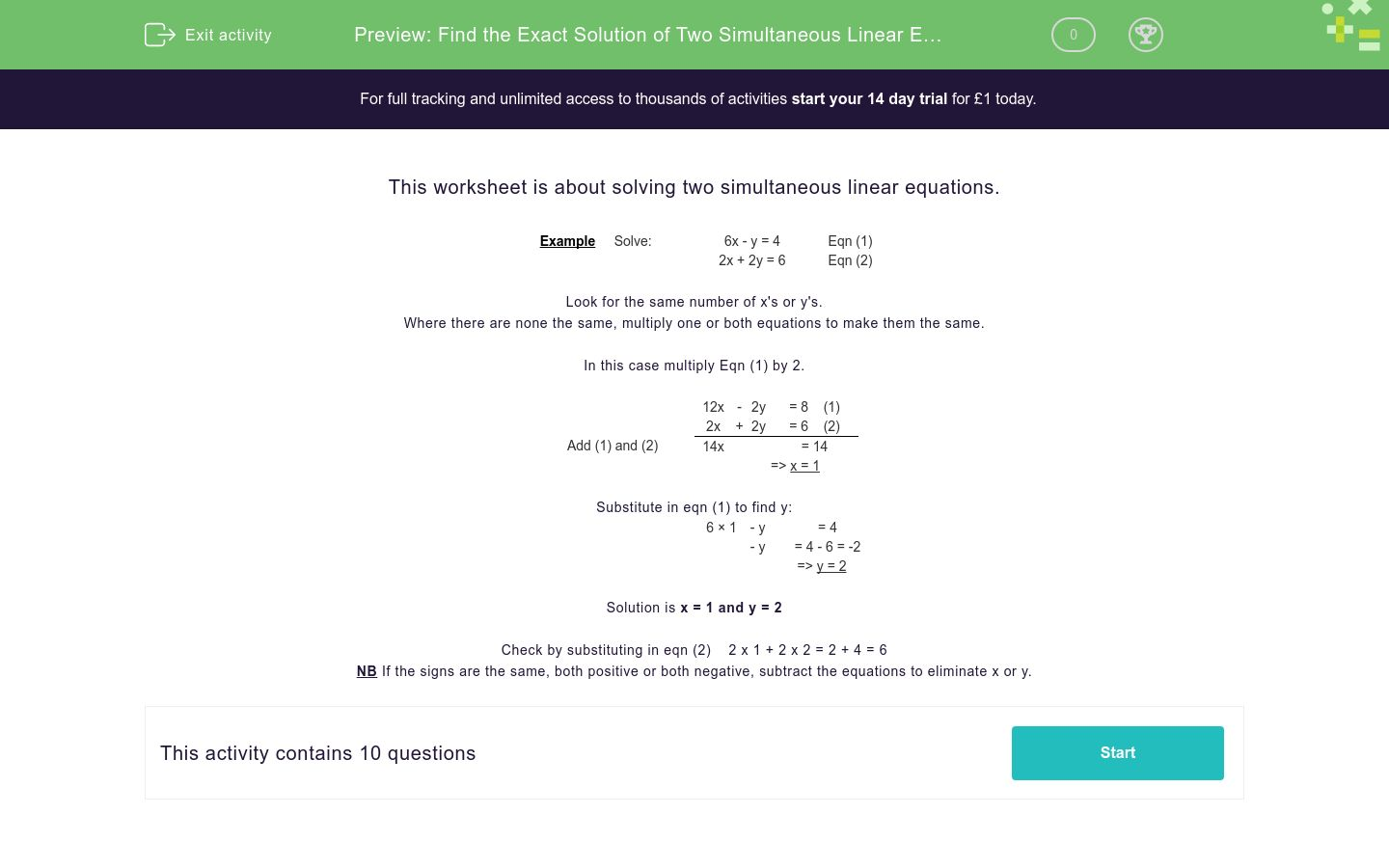# Find the Exact Solution of Two Simultaneous Linear Equations

In this worksheet, students solve simultaneous linear equations.Key stage:  KS 4

Curriculum topic:  Algebra

Curriculum subtopic:  Solve Simultaneous Equations in 2 Variables

Difficulty level:### QUESTION 1 of 10

This worksheet is about solving two simultaneous linear equations.

 Example     Solve: 6x - y = 4 Eqn (1) 2x + 2y = 6 Eqn (2)

Look for the same number of x's or y's.

Where there are none the same, multiply one or both equations to make them the same.

In this case multiply Eqn (1) by 2.

 12x - 2y = 8    (1) 2x + 2y = 6    (2) Add (1) and (2) 14x = 14 => x = 1

Substitute in eqn (1) to find y:

 6 × 1 - y = 4 - y = 4 - 6 = -2 => y = 2

Solution is x = 1 and y = 2

Check by substituting in eqn (2)    2 x 1 + 2 x 2 = 2 + 4 = 6

NB If the signs are the same, both positive or both negative, subtract the equations to eliminate x or y.

Solve the following pair of simultaneous equations:

 8x + 5y = 9 2x + y = 1

x = y =
 x y 1 -1 5 -2

Solve the following pair of simultaneous equations:

 5x + 8y = -6 5x + y = 8

x = y =
 x y 2 -1 5 -2

Solve the following pair of simultaneous equations:

 4x + 2y = 0 x + y = 10

x = y =
 x y 20 -10 5 -20

Solve the following pair of simultaneous equations:

 3x + 8y = 10 2x - 8y = 0

x = y =
 x y 2 0.5 1.5 -2

Solve the following pair of simultaneous equations:

 4x + y = 6 5x + y = 9

x = y =
 x y 2 -5 3 -6

Solve the following pair of simultaneous equations:

 4x + 6y = 1 x - 6y = 4

x = y =
 x y -0.83 -0.5 1 -6

Solve the following pair of simultaneous equations:

 5x + 4y = 2 x + y = 4

x = y =
 x y -15 -8 18 -14

Solve the following pair of simultaneous equations:

 5x + 2y = 1 2x + y = -8

x = y =
 x y -15 - 42 18 17

Solve the following pair of simultaneous equations:

 x + 4y = 7 x - 4y = -5

x = y =
 x y -1 1 1.5 1.7

Solve the following pair of simultaneous equations:

 3x + 7y = -2 2x + y = -5

x = y =
 x y -1 1 - 3 1.7
• Question 1

Solve the following pair of simultaneous equations:

 8x + 5y = 9 2x + y = 1

x = y =
 x y 1 -1 5 -2
• Question 2

Solve the following pair of simultaneous equations:

 5x + 8y = -6 5x + y = 8

x = y =
 x y 2 -1 5 -2
• Question 3

Solve the following pair of simultaneous equations:

 4x + 2y = 0 x + y = 10

x = y =
 x y 20 -10 5 -20
• Question 4

Solve the following pair of simultaneous equations:

 3x + 8y = 10 2x - 8y = 0

x = y =
 x y 2 0.5 1.5 -2
• Question 5

Solve the following pair of simultaneous equations:

 4x + y = 6 5x + y = 9

x = y =
 x y 2 -5 3 -6
• Question 6

Solve the following pair of simultaneous equations:

 4x + 6y = 1 x - 6y = 4

x = y =
 x y -0.83 -0.5 1 -6
• Question 7

Solve the following pair of simultaneous equations:

 5x + 4y = 2 x + y = 4

x = y =
 x y -15 -8 18 -14
• Question 8

Solve the following pair of simultaneous equations:

 5x + 2y = 1 2x + y = -8

x = y =
 x y -15 - 42 18 17
• Question 9

Solve the following pair of simultaneous equations:

 x + 4y = 7 x - 4y = -5

x = y =
 x y -1 1 1.5 1.7
• Question 10

Solve the following pair of simultaneous equations:

 3x + 7y = -2 2x + y = -5

x = y =